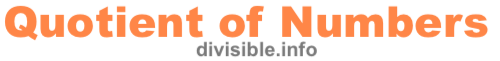What is the Quotient of 12 and 27?What is the quotient of 12 and 27? This is easy to answer if you know the different parts of a division problem.

Division is breaking something up into equal parts. The Dividend is the whole you start with, the Divisor is what you use to divide up the whole, and the Quotient is the answer like this:

Dividend ÷ Divisor = Quotient

So when you ask, "What is the quotient of 12 and 27?", it makes sense that 12 is the Dividend, 27 is the Divisor, and you want to know the Quotient. Thus, the answer is:

12 ÷ 27 = 0.444444

Note: The answer (quotient) is rounded up to six decimal points if necessary.

Quotient of Numbers Calculator
Please enter another x and y to get the quotient for those numbers:

and

What is the Quotient of 12 and 28?
Go here to get the quotient for the next numbers on our list.